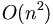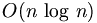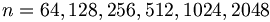Difference between revisions of "Fast Fourier Transform"

Fast Fourier Transform (FFT) is an efficient algorithm for calculating the discrete fourier transform (DFT). It reduces the execution time by hundreds in some cases. Whereas DFT takes an order of$O(n^2)\,$ computations, FFT takes an order of$O(n\,\log\,n)$, and is definitely the preferred algorithm to be used in all applications in terms of computational complexity. The FFT in most implementations consistent of samples that are exactly a power of 2, this is commonly known as a FFT Radix 2 algorithm where$n = 64,128,256,512,1024,2048$ etc.